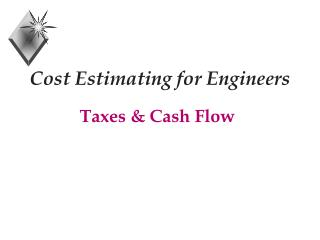DownloadDownload PresentationCost Estimating for Engineers

# Cost Estimating for Engineers

Télécharger la présentation## Cost Estimating for Engineers

- - - - - - - - - - - - - - - - - - - - - - - - - - - E N D - - - - - - - - - - - - - - - - - - - - - - - - - - -
##### Presentation Transcript

1. Cost Estimating for Engineers Taxes & Cash Flow

2. Taxable Income + Gross Income - Depreciation Allowance - Interest on Borrowed Money - Other Tax Exemptions = Taxable Income

3. Corporate Tax Rate

4. Corporate Tax Ex: Suppose K-Corp earns \$5,000,000 in revenue above manufacturing and operations cost. Suppose further that depreciation costs total \$800,000 and interest paid on short and long term debt totals \$1,500,000. Compute the tax paid.

5. After Tax Cash Flow + Gross Income - Interest = Before Tax Cash Flow - Tax = After Tax Cash Flow

6. After Tax Cash Flow Ex: Suppose K-Corp earns \$5,000,000 in revenue above manufacturing and operations cost. Suppose further that depreciation costs total \$800,000 and interest paid on short and long term debt totals \$1,500,000. Compute the after tax cash flow.

7. After Tax Cash Flow Gross Income \$ 5,000,000 Depreciation - 800,000 Interest - 1,500,000 Before Tax Cash Flow \$ 2,700,000

8. After Tax Cash Flow Formulas BTCF = Before Tax Cash Flow = Revenues - Expenses TI = Taxable Income = Cash Flow - Interest - Depreciation Tax = TI * Tax Rate ATCF = After Tax Cash Flow = BTCF - Tax

9. Ex: After Tax Cash Flow

10. Borrowed Money

11. Class Problem A company plans to invest in a water purification system (5 year property) requiring \$800,000 capital. The system will last 7 years with a salvage of \$100,000. The before-tax cash flow for each of years 1 to 6 is \$200,000. Regular MACRS depreciation is used; the applicable tax rate is 34%. Construct a table showing each of the following for each of the 7 years.

12. Solution

13. Solution